# NCERT Solutions for Class 7 Maths Chapter 2 Fractions and Decimals Ex 2.2

NCERT Solutions for Class 7 Maths Chapter 2 Fractions and Decimals Exercise 2.2
Ex 2.2 Class 7 Maths Question 1.
Which of the drawings (a) to (d) show.
$$\begin{array}{ll}{(i) 2 \times \frac{1}{5}} & {(i i) 2 \times \frac{1}{2}} \\ {(i i i) 3 \times \frac{2}{3}} & {(i v) 3 \times \frac{1}{4}}\end{array}$$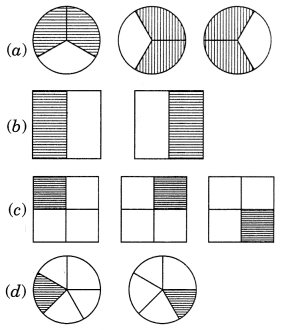Solution:
(i) $$2 \times \frac{1}{5}$$ represents drawing (d)
(ii) $$2 \times \frac{1}{2}$$ represents drawing (b)
(iii) $$3 \times \frac{2}{3}$$ represents drawing (a)
(ic) $$3 \times \frac{1}{4}$$ represents drawing (c)

Ex 2.2 Class 7 Maths Question 2.
Some pictures (a) to (c) are given below. Tell which of them show: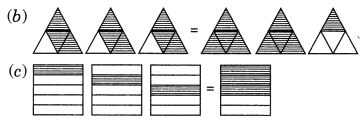Solution:
(i) $$3 \times \frac{1}{5}=\frac{3}{5}$$ represents figure (c)
(ii) $$2 \times \frac{1}{3}=\frac{2}{3}$$ represents figure (a)
(iii) $$3 \times \frac{3}{4}=2 \frac{1}{4}$$ represents figure (b)

Ex 2.2 Class 7 Maths Question 3.
Multiply and reduce to lowest form and convert into a mixed fraction: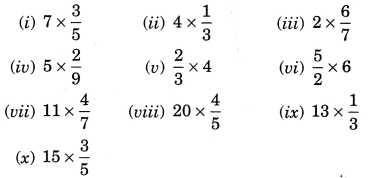Solution: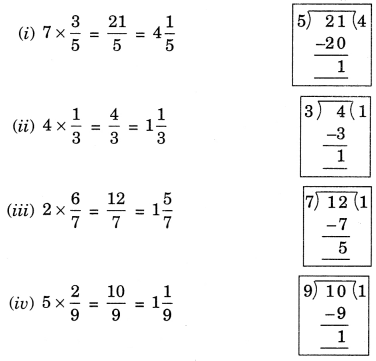Ex 2.2 Class 7 Maths Question 4.
(i) $$\frac{1}{2}$$ of the circles in box (a)
(ii) $$\frac{2}{3}$$ of the circles in box (b)
(iii) $$\frac{3}{5}$$ of the circles in box (c)Solution: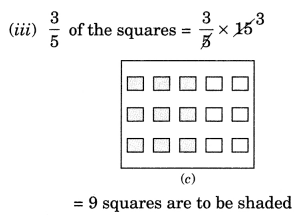Ex 2.2 Class 7 Maths Question 5.
Find:Solution: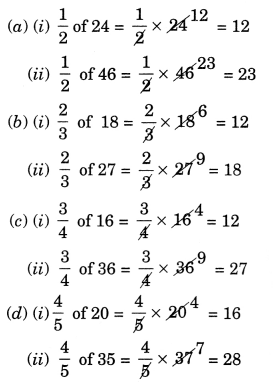Ex 2.2 Class 7 Maths Question 6.
Multiply and express as a mixed fraction.Solution:Ex 2.2 Class 7 Maths Question 7.
Find:Solution:Ex 2.2 Class 7 Maths Question 8.
Vidya and Pratap went for a picnic. Their mother gave them a water bottle that contained 5 litres of water. Vidya consumed $$\frac{2}{5}$$ of the water. Pratap consumed the remaining water.
(i) How much water did Vidya drink?
(ii) What fraction of the total quantity of water did Pratap drink?
Solution:
(i) Water consumed by Vidya = $$\frac{2}{5}$$ of 5 litres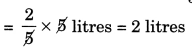Water consumed by Patap
= 5 litres – 2 litres = 3 litres
(ii) Fraction of water consumed by Pratap
= $$\frac{3}{5}$$ litres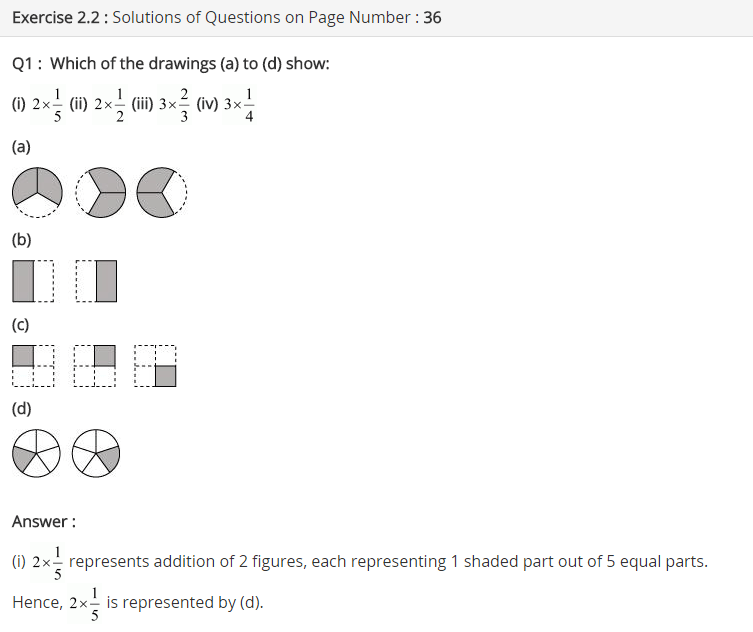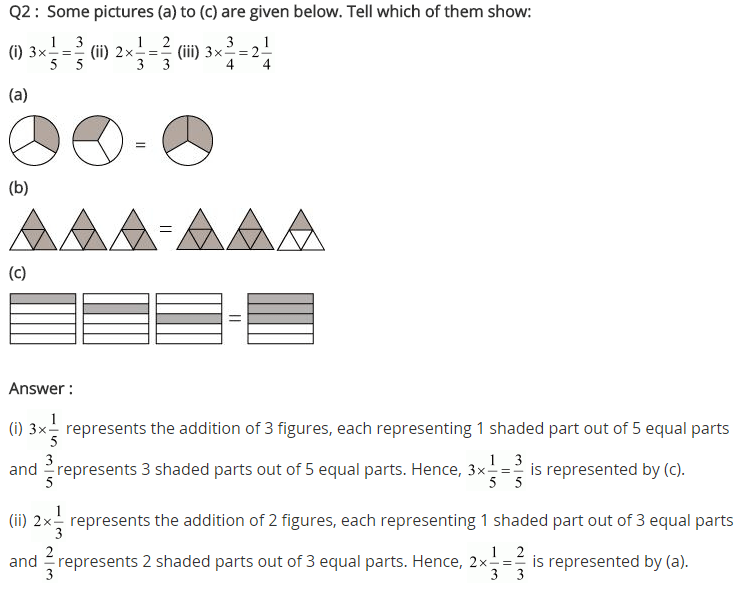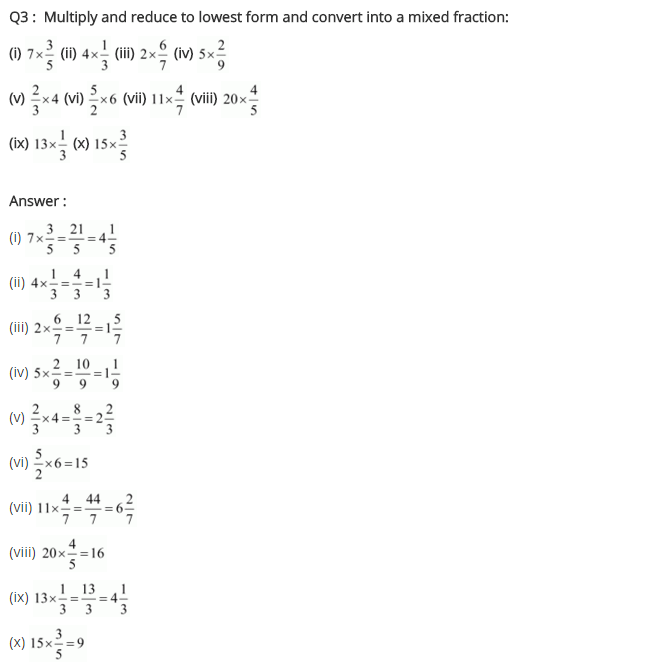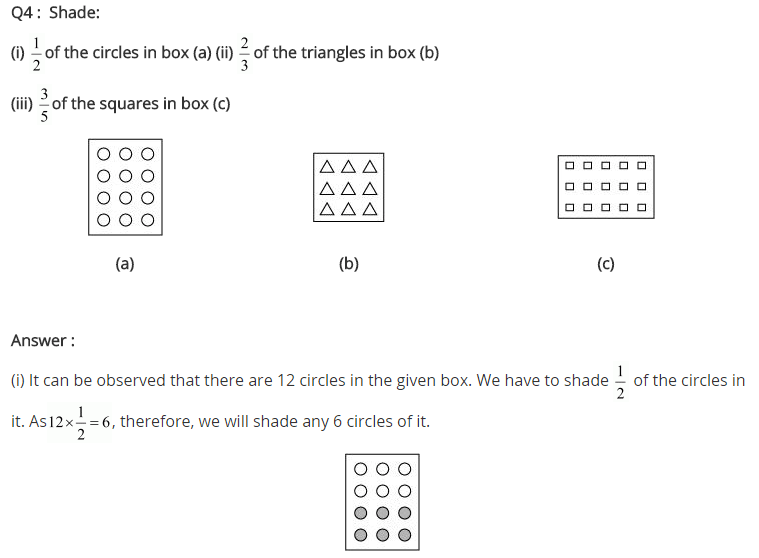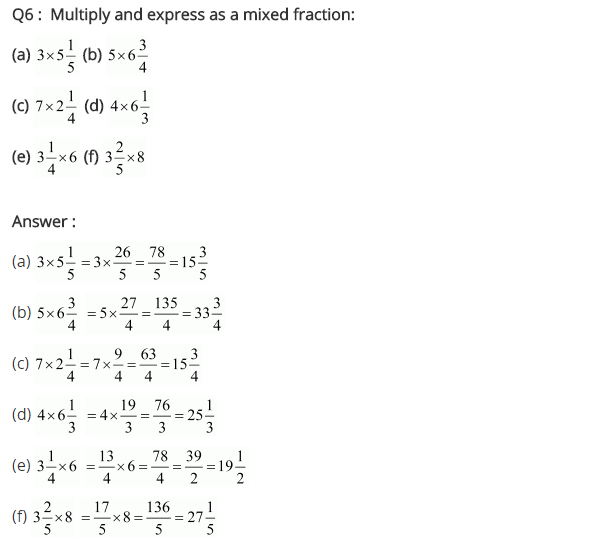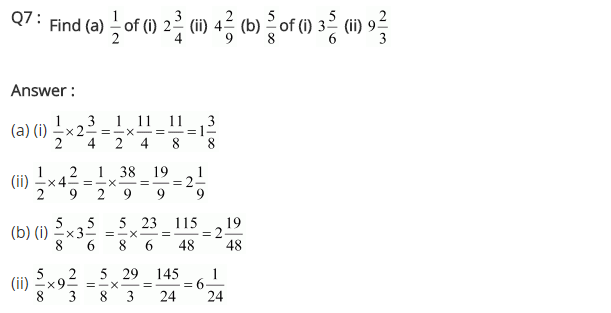+